# Parabolic coordinates

Jump to: navigation, search

The numbersandrelated to rectangular Cartesian coordinatesandby the formulas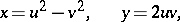whereand. The coordinate lines are two systems of mutually orthogonal parabolas with oppositely-directed axes.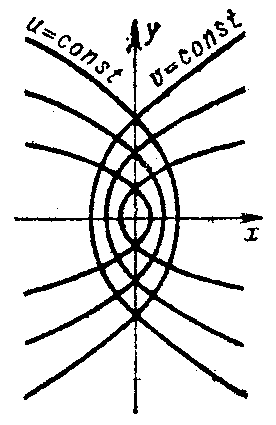Figure: p071170a

The Lamé coefficients (or scale factors) are given by: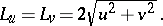The area element is given by:The fundamental operators of vector analysis are given by: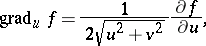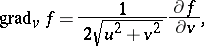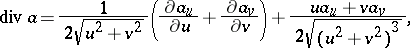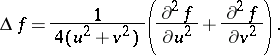In parabolic coordinates the Laplace equation allows separation of variables.

#### Comments

Using complex functions the coordinate transformation can be described by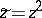, where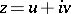and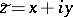.

For parabolic coordinates in space see [a1].